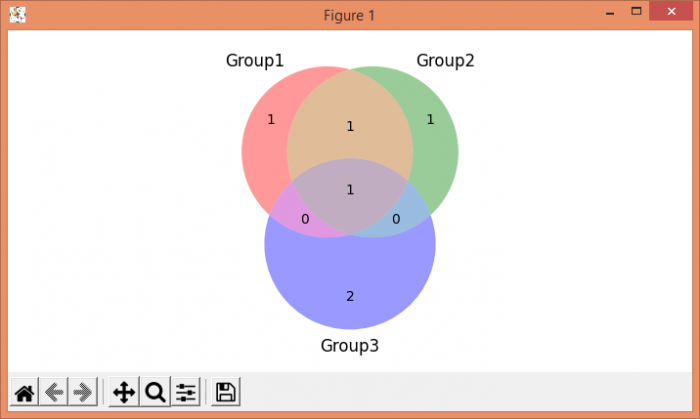# Python Matplotlib Venn diagram

MatplotlibServer Side ProgrammingProgramming

To plot a Venn diagram, first install Venn diagram using command "pip install matplotlib-venn". Using venn3, plot a 3-set area-weighted Venn diagram.

## Steps

• Create 3 sets.

• Using venn3, make a Venn diagram.

• To display the figure, use show() method.

## Example

import matplotlib.pyplot as plt
from matplotlib_venn import venn3
plt.rcParams["figure.figsize"] = [7.00, 3.50]
plt.rcParams["figure.autolayout"] = True
set1 = {'A', 'B', 'C'}
set2 = {'A', 'B', 'D'}
set3 = {'A', 'E', 'F'}
venn3([set1, set2, set3], ('Group1', 'Group2', 'Group3'))
plt.show()

## Output